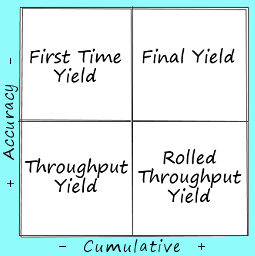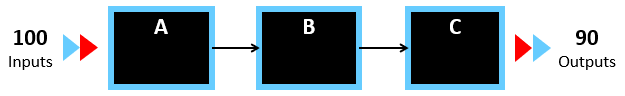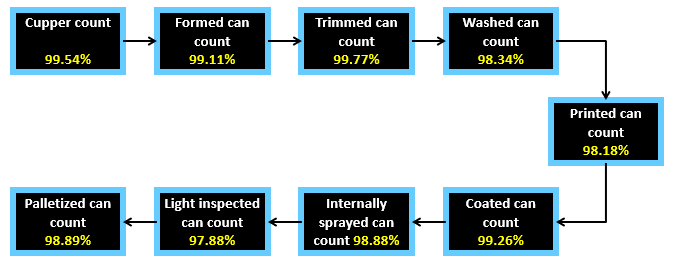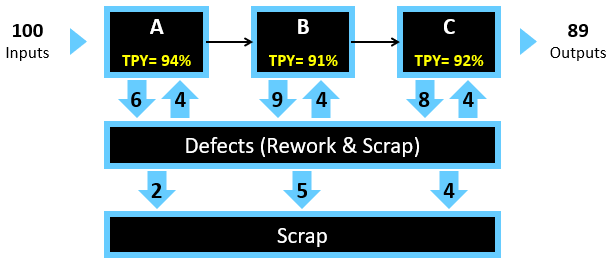By the CIToolkit content team   |   4 minutes read

# Process Yield MeasuresAn ideal process must produce without defects and without rework. To expose these unnecessary and costly inefficiencies, you should have appropriate performance metrics to measure process yield, or otherwise, the true process yield might be underestimated. Process yield measures should be able to expose even the smallest inefficiencies in a process, which will enable operations to understand their true process yield in order to set realistic improvement targets.

A best practice is to use a process map as a guide in the process yield evaluation.

Many companies use two measures of process yield: first time yield and final yield. These companies often find they have high yield rates and assume their processes are performing efficiently and effectively. Both metrics represent the classic approach for calculating process yield and don’t account for the hidden factory such as rework and delays.

First Time Yield (FTY) is simply obtained by dividing the good product units by the number of total units entered the process at a given process step. Such good product units may include reworked units. For example, first time yield at a given process step which has produced 90 good units from 100 processed units would be 90 percent.First time yield is not sensitive to product complexity and only looks at the volume of the produced units. Corrective actions are often taken on spot when mistakes are discovered, and rework are not recorded in quality logs making the process yield rate looks better than what it really is.

Final Yield (FY) is another widely used metric that is easy to calculate using readily available data. It is obtained by counting the good units that made it through until the last step divided by the total number of units that entered the process. In other words, if the amount of units at the end of the process equals to what they were at the beginning, then the final yield would be 100 percent.The final yield for this 3-step process equals to 90%

Throughput Yield (TPY) on the other hand is a good metric to start with for measuring process yield. It is the probability that a product or service unit will pass through a given process step defect-free. It only considers the good units that passed through a process step right the first time and error-free. In other words, a reworked unit that passed the process step will not be considered in the calculation of throughput yield.

Another metric that considers the entire process flow from beginning to end is the Rolled Throughput Yield (RTY). It quantifies the cumulative effects of inefficiencies found throughout the process and provides better insight of error and rework rates. It is calculated by multiplying the individual throughput yield values of all process steps:

Rolled Throughput Yield (RTY) = Throughput Yield of process step 1 * Throughput Yield of process step 2 * … * Throughput Yield of process N.

Rolled throughput yield allows companies to be much more accurate when assessing the performance of their industrial or commercial processes because calculations are done at each process step. Additionally, it should be noted that rolled throughput yield is substantially less than final yield, and companies that calculated their final yield at 90 percent might find their rolled throughput yield less than 50 percent.

## Example – High Volume Process

The following is a can-making process map. The probability of manufacturing a can that meets all performance standards would be 90.28% (i.e. RTY = 90.28%).## Example – Low Complexity Process

Consider a process that has 3 steps performing at a 0.94, 0.91 and 0.92 respectively. Suppose that 100 units entered the process and only 89 were good units. Find the final yield and the rolled throughput yield for the overall process.Answer: Final yield would be 89%, and rolled throughput yield would be: 0.94 x 0.91 x 0.92 = 78.7%. This means that even if the 3 processes are performing fairly well, one out of every 5 units will not make it through the process without being scrapped or reworked.

Other Formats

Related Articles

Related Templates